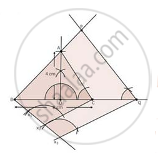Share

# Construct an Isosceles Triangle Whose Base is 8 Cm and Altitude 4 Cm and Then Another Triangle Whose Sides Are 3/2 Times the Corresponding Sides of the Isosceles Triangle. - CBSE Class 10 - Mathematics

#### Question

Construct an isosceles triangle whose base is 8 cm and altitude 4 cm and then another triangle whose sides are 3/2 times the corresponding sides of the isosceles triangle.

#### SolutionSteps to construct

1. Draw a line segment BC of 8 cm

2. Take a mid point D of BC

3. At D, draw an angler of 90°

4.With centre D,Radius 4cm, draw an arc which intersect line of angle at A

5. Join AB and AC

6. At B, draw an angle CBX of any measure

7. Starting from B, cut there equal parts on BX such that BX1 = X1X2 = X2X3

8. Join X2C

9. Through X3, Draw X3Q || X2C

10. Through Q, Draw QP || CA

∴ ΔPBQ ∼ ΔABC

Is there an error in this question or solution?

#### APPEARS IN

Solution Construct an Isosceles Triangle Whose Base is 8 Cm and Altitude 4 Cm and Then Another Triangle Whose Sides Are 3/2 Times the Corresponding Sides of the Isosceles Triangle. Concept: Division of a Line Segment.
S